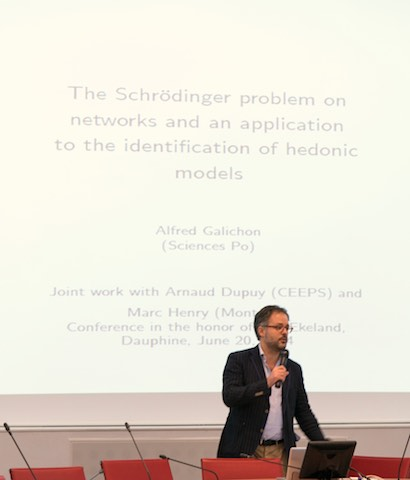# Best of my (scientific) bookshelf

Without any logical order or any explanation of my picks, here is a selection of my very favorite books:

• Villani, C. (2003). Topics in Optimal Transportation. AMS.
• Vohra, R. (2011). Mechanism design. A linear programming approach. Cambridge.
• Vohra, R. (2004). Advanced Mathematical Economics. Routledge.
• Frankel, T. (2012). The geometry of physics. An introduction. Cambridge.
• Gale, D. (1960). The theory of linear economic models. Chicago.
• Burkard, R. Dell’Amico, M., Martello, S. (2012) Assignment Problems. SIAM.
• Henry-Labordere, P. (2008). Analysis, Geometry, and Modeling in Finance: Advanced methods in option pricing. Chapman & Hall.
• Roth, A. and Sotomayor, M. (1990). Two-sided matching. A Study in Game-Theoretic Modeling and Analysis. Cambridge.
• Border, K. (1989). Fixed Point Theorems with Applications to Economics and Game Theory. Cambridge.
• Krishna, V. (2010). Auction Theory. Second edition. Elsevier.
• Hiriart-Urruty, J.-B., and Claude Lemaréchal, C. (2004). Fundamentals of Convex Analysis. Springer.
• Aubin, J.-P., and Ekeland, I. (2006). Applied nonlinear analysis. Dover.
• Grady, L., and Polimeni, J. (2010). Discrete Calculus: Applied Analysis on Graphs for Computational Science. Springer.
• Bertsekas, D. (1998). Network Optimization: Continuous and Discrete Models (Optimization, Computation, and Control). Athena Scientific.
• Bobzin, H. (2008). Principles of Network Economics. Springer.
• Rheinboldt, W. (1987). Methods for Solving Systems of Nonlinear Equations. SIAM.
• Bhatia, R. (2011). Matrix Analysis. Springer.
• Horn, R. and Johnson, C. (1994). Topics in Matrix Analysis. Cambridge.

# Optimal Transport Methods in Economics

Princeton University Press

This text provides an introduction to the theory of optimal transport, with a focus on applications to microeconomics and econometrics. It intends to cover the basic results in optimal transport, in connection with linear programming, network flow problems, convex analysis, and computational geometry. Several applications to various fields in economic analysis (econometrics, family economics, labor economics and contract theory) are provided.
The book is available on Amazon.com here.
The book webpage on the publisher’s site is here.
The programming examples and solution programs to the exercises are implemented in R and are available here.
An erratum can be found here here

# Pictures

Conference in the honor of Ivar Ekeland’s 70th birthday, Paris, June 2014 (Photo: Julien Ling)